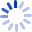# Statistical inference

This book builds theoretical statistics from the first principles of probability theory. Starting from the basics of probability, the authors develop the theory of statistical inference using techniques, definitions, and concepts that are statistical and are natural extensions and consequences of previous concepts. Intended for first-year graduate students, this book can be used for students majoring in statistics who have a solid mathematics background. It can also be used in a way that stresses the more practical uses of ...

##### Cash for Textbooks